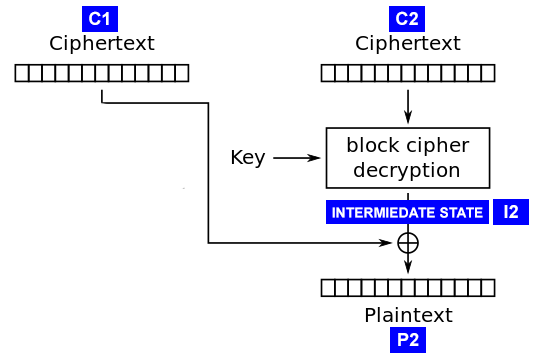对称加密与攻击案例分析

2019-06-02对称加密

• 流加密（Stream Ciphers）：逐字节加密数据
• 块加密（Block Ciphers）：逐块加密数据

0 xor 0 = 0
0 xor 1 = 1
1 xor 0 = 1
1 xor 1 = 0

块加密

• ‘AAAAAAA’ + ‘\x01’
• ‘AAAAAA’ + ‘\x02\x02’
• ‘AA’ + ‘\x06’ * 6
• ‘A’ + ‘\x07’ * 7
• ‘\x08’ * 8

加密模式

ECB

ECB全称为Electronic CodeBook，是块加密中比较简单的加密模式。在ECB模式中，每一块明文数据都被独立地进行加密来生成加密块。这意味着如果你发现两个加密块有相同的内容，那么就可以确定这两个加密块的原文也是相同的。CBC

CBC全称为Cipher-Block Chaining，算是最常见的块加密模式了。在CBC模式中，每个明文块都会在加密前被使用前一个明文块的秘文进行异或；解密过程则正好相反。其中第一个明文块会被使用IV即初始化向量进行异或。常见攻击

流加密重用攻击

E(A) = A xor C
E(B) = B xor C

E(A) xor E(B) = (A xor C) xor (B xor C) = A xor B xor C xor C = A xor B

A = E(A) xor E(B) xor B

\$ cat 1.txt
hello
\$ cat 2.txt
world

\$ openssl rc4 -nosalt -in 1.txt > 1.enc
\$ openssl rc4 -nosalt -in 2.txt > 2.enc

#!/usr/bin/env python3

with open(file, 'rb') as f:
print('loaded', len(data), 'bytes from', file)
return data

def xor(lhs, rhs):
return bytes(a ^ b for a, b in zip(lhs, rhs))

#  A = load('./1.txt')

print('E(A) =', A_enc)
print('E(B) =', B_enc)
print('B =', B)
print('A =', xor(xor(B, B_enc), A_enc))

\$ python3 stream.py
loaded 6 bytes from ./1.enc
loaded 6 bytes from ./2.txt
loaded 6 bytes from ./2.enc
E(A) = b'\xa1\xb1`\x1b\xa7\x97'
E(B) = b'\xbe\xbb~\x1b\xac\x97'
B = b'world\n'
A = b'hello\n'

ECB块重排攻击

=pan@@@@

=pan@@@@

\$ openssl des-ecb -nosalt -in admin.txt > admin.enc
00000000: 0293 07cd 88f3 026e c61e 1284 1a6e 6853  .......n.....nhS
00000010: e0b2 7169 3ee4 0b9a                      ..qi>...

00000000: c61e 1284 1a6e 6853 0293 07cd 88f3 026e  .....nhS.......n
00000010: e0b2 7169 3ee4 0b9a                      ..qi>...
\$ openssl des-ecb -nosalt -d -in admin1.enc

最后一字节• 将C1前15字节随机设置，第16字节设置为’\x00’
• 将修改后的密文块发送给服务器解密

P2 = I2 xor C1

1. 返回填充不合法。此时P2未知。
2. 返回填充合法。此时P2肯定为0x01，因为只有这样才能出现合法的填充。

P2 = I2 xor C1
I2 = P2 xor C1 = 0x01 xor 0x26 = 0x27

P2 = 0x27 xor C1

下一个字节

• 将C1前14字节设置为随机值
• C1设置为0x00
• C1设置为能令P2 = 0x02的值
P2 = I2 xor C1
C1 = P2 xor I2 = 0x02 xor 0x27 = 0x25

P2 = I2 xor C1 = 0x02
I2 = P2 xor C1 = 0x02 xor 0x68 = 0x6A

示例

#!/usr/bin/env python3
import binascii
import string
import random

from Crypto.Cipher import AES

db = {}
BSIZE = 16
secret = b'\x26' * BSIZE

def get_iv():
return b'\x00' * BSIZE

def decrypt(data):
data = data.encode()
data = binascii.unhexlify(data)
iv = data[:BSIZE]
engine = AES.new(key=secret, mode=AES.MODE_CBC, iv=iv)
data = data[BSIZE:]
data = engine.decrypt(data)
data = unpad(data, BSIZE)
return data.decode()

def encrypt(data):
data = data.encode()
iv = get_iv()
engine = AES.new(key=secret, mode=AES.MODE_CBC, iv=iv)
return binascii.hexlify(iv + engine.encrypt(pad(data, BSIZE))).decode()

@app.route('/dec/<data>')
def dec(data):
# print('dec:', data)
try:
key = decrypt(data)
except Exception as e:
return 'Error: ' + str(e)
if key not in db:
return 'Error: invalid key'
return db[key]

@app.route('/enc/<key>')
def enc(key):
db[key] = 'valid'
return encrypt(key)

app.run(debug=False)

\$ curl http://localhost:5000/enc/See_you_in_Red_Square_at_4_pm

valid

Error: Padding is incorrect.

block = '00000000000000000000000000000000'
block = 'dac1d6573e7c26d533ffc3cbc23a8455'

I2 = _C1 ^ _P2 = 0x3b ^ 0x01 = 0x3a

P2 = I2 xor C1 = 0x3a ^ 0x39 = 0x03

#!/usr/bin/env python3
import time
import requests
import binascii

url = 'http://localhost:5000/dec/'
BSIZE = 16

def test(data):
r = requests.get(url + data)
return r.text

b = binascii.unhexlify(data)
nblocks = int(len(b) / BSIZE)
blocks = []
print('nblocks:', nblocks)
for i in range(nblocks):
blk = b[i*BSIZE: (i+1)*BSIZE]
print(f'block[{i}] =', binascii.hexlify(blk))
blocks.append(blk)
print('iv:', b[:BSIZE])

blockID = -1
prevID = blockID - 1

print(f'decrypting block[{blockID}], prev =', binascii.hexlify(blocks[prevID]))

plaintext = bytearray(16)
inter = bytearray(16)
for byteIdx in range(BSIZE-1, -1, -1):
prevBlock = bytearray(blocks[prevID])
print(f'mutating block[{prevID}][{byteIdx}]')
origin = prevBlock[byteIdx]
padValue = BSIZE - byteIdx
# 将byteIdx之前的值可以任意随机设置
for i in range(byteIdx):
prevBlock[i] = 0x11
for i in range(byteIdx + 1, BSIZE):
prevBlock[i] = inter[i] ^ padValue
print('begin:', prevBlock.hex())
found = False
for val in range(0x100):
prevBlock[byteIdx] = val
_blocks = blocks.copy()
_blocks[prevID] = bytes(prevBlock)
# print(f'testing', binascii.hexlify(prevBlock), '->', resp, end='\r')
if 'incorrect' in resp:
continue
i2 = padValue ^ val
p2 = origin ^ i2
inter[byteIdx] = i2
plaintext[byteIdx] = p2
print(f'found c={val}, i={padValue}^{val}={i2}, o={origin}, p={p2}')
found = True
break
print('Error: no valid value found')
break
print('plaintext =', plaintext)

nblocks: 3
block = b'00000000000000000000000000000000'
block = b'dac1d6573e7c26d533ffc3cbc23a8455'
iv: b'\x00\x00\x00\x00\x00\x00\x00\x00\x00\x00\x00\x00\x00\x00\x00\x00'
decrypting block[-1], prev = b'c8ab1c881b40d54d81d1efab429ad239'
mutating block[-2]
begin: 11111111111111111111111111111139
found c=59, i=1^59=58, o=57, p=3
mutating block[-2]
begin: 1111111111111111111111111111d238
found c=211, i=2^211=209, o=210, p=3
mutating block[-2]
found c=154, i=3^154=153, o=154, p=3
mutating block[-2]
begin: 111111111111111111111111429dd53e
found c=43, i=4^43=47, o=66, p=109
mutating block[-2]
begin: 1111111111111111111111ab2a9cd43f
found c=222, i=5^222=219, o=171, p=112
mutating block[-2]
begin: 11111111111111111111efdd299fd73c
found c=182, i=6^182=176, o=239, p=95
mutating block[-2]
begin: 111111111111111111d1b7dc289ed63d
found c=226, i=7^226=229, o=209, p=52
mutating block[-2]
begin: 111111111111111181edb8d32791d932
found c=214, i=8^214=222, o=129, p=95
mutating block[-2]
begin: 111111111111114dd7ecb9d22690d833
found c=48, i=9^48=57, o=77, p=116
mutating block[-2]
found c=190, i=10^190=180, o=213, p=97
mutating block[-2]
begin: 111111111140bf32d5eebbd02492da31
found c=20, i=11^20=31, o=64, p=95
mutating block[-2]
begin: 111111111b13b835d2e9bcd72395dd36
found c=114, i=12^114=126, o=27, p=101
mutating block[-2]
begin: 111111887312b934d3e8bdd62294dc37
found c=247, i=13^247=250, o=136, p=114
mutating block[-2]
begin: 11111cf47011ba37d0ebbed52197df34
found c=115, i=14^115=125, o=28, p=97
mutating block[-2]
begin: 11ab72f57110bb36d1eabfd42096de35
found c=209, i=15^209=222, o=171, p=117
mutating block[-2]
begin: c8ce6dea6e0fa429cef5a0cb3f89c12a
found c=169, i=16^169=185, o=200, p=113
plaintext = bytearray(b'quare_at_4_pm\x03\x03\x03')